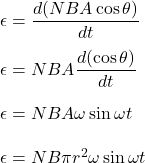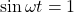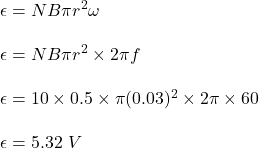## A 10-turn conducting loop with a radius of 3.0 cm spins at 60 revolutions per second in a magnetic field of 0.50T. The maximum emf generated

Question

A 10-turn conducting loop with a radius of 3.0 cm spins at 60 revolutions per second in a magnetic field of 0.50T. The maximum emf generated is:

in progress 0
1 month 2021-08-12T08:08:42+00:00 1 Answers 4 views 0

Maximum emf = 5.32 V

Explanation:

Given that,

Number of turns, N = 10

Radius of loop, r = 3 cm = 0.03 m

It made 60 revolutions per second

Magnetic field, B = 0.5 T

We need to find maximum emf generated in the loop. It is based on the concept of Faraday’s law. The induced emf is given by :For maximum emf,So,So, the maximum emf generated in the loop is 5.32 V.